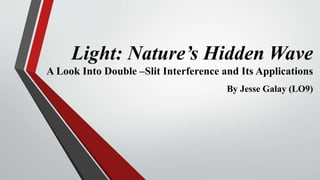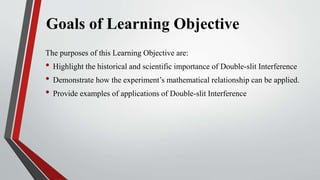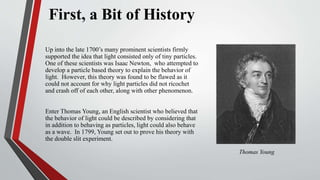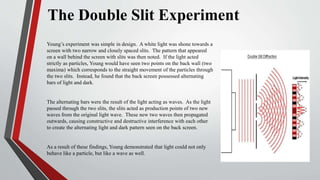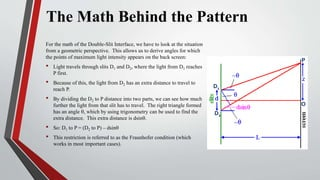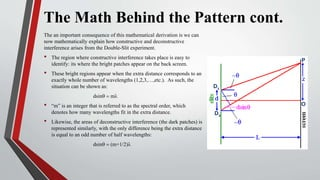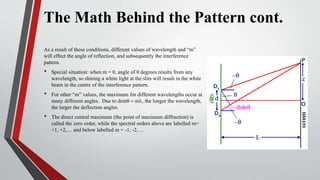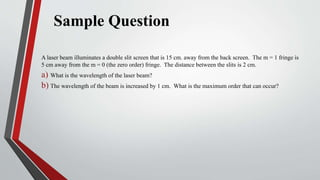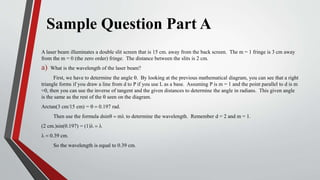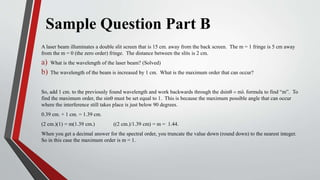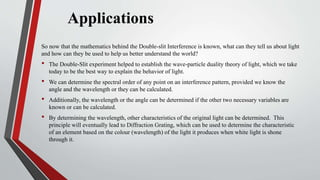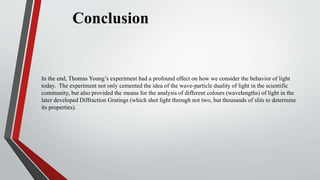1 of 12

### Lo9 physics

1. Light: Nature’s Hidden Wave A Look Into Double –Slit Interference and Its Applications By Jesse Galay (LO9)
2. Goals of Learning Objective The purposes of this Learning Objective are: • Highlight the historical and scientific importance of Double-slit Interference • Demonstrate how the experiment’s mathematical relationship can be applied. • Provide examples of applications of Double-slit Interference
3. First, a Bit of History Up into the late 1700’s many prominent scientists firmly supported the idea that light consisted only of tiny particles. One of these scientists was Isaac Newton, who attempted to develop a particle based theory to explain the behavior of light. However, this theory was found to be flawed as it could not account for why light particles did not ricochet and crash off of each other, along with other phenomenon. Enter Thomas Young, an English scientist who believed that the behavior of light could be described by considering that in addition to behaving as particles, light could also behave as a wave. In 1799, Young set out to prove his theory with the double slit experiment. Thomas Young
4. The Double Slit Experiment Young’s experiment was simple in design. A white light was shone towards a screen with two narrow and closely spaced slits. The pattern that appeared on a wall behind the screen with slits was then noted. If the light acted strictly as particles, Young would have seen two points on the back wall (two maxima) which corresponds to the straight movement of the particles through the two slits. Instead, he found that the back screen possessed alternating bars of light and dark. The alternating bars were the result of the light acting as waves. As the light passed through the two slits, the slits acted as production points of two new waves from the original light wave. These new two waves then propagated outwards, causing constructive and destructive interference with each other to create the alternating light and dark pattern seen on the back screen. As a result of these findings, Young demonstrated that light could not only behave like a particle, but like a wave as well.
5. The Math Behind the Pattern For the math of the Double-Slit Interface, we have to look at the situation from a geometric perspective. This allows us to derive angles for which the points of maximum light intensity appears on the back screen: • Light travels through slits D1 and D2, where the light from D1 reaches P first. • Because of this, the light from D2 has an extra distance to travel to reach P. • By dividing the D2 to P distance into two parts, we can see how much further the light from that slit has to travel. The right triangle formed has an angle q, which by using trigonometry can be used to find the extra distance. This extra distance is dsinq. • So: D1 to P = (D2 to P) – dsinq • This restriction is referred to as the Fraunhofer condition (which works in most important cases). D1 D2 P O
6. The Math Behind the Pattern cont. The an important consequence of this mathematical derivation is we can now mathematically explain how constructive and deconstructive interference arises from the Double-Slit experiment. • The region where constructive interference takes place is easy to identify: its where the bright patches appear on the back screen. • These bright regions appear when the extra distance corresponds to an exactly whole number of wavelengths (1,2,3,…,etc.). As such, the situation can be shown as: dsinq = ml • “m” is an integer that is referred to as the spectral order, which denotes how many wavelengths fit in the extra distance. • Likewise, the areas of deconstructive interference (the dark patches) is represented similarly, with the only difference being the extra distance is equal to an odd number of half wavelengths: dsinq = (m+1/2)l D1 D2 P O
7. The Math Behind the Pattern cont. As a result of these conditions, different values of wavelength and “m” will effect the angle of reflection, and subsequently the interference pattern. • Special situation: when m = 0, angle of 0 degrees results from any wavelength, so shining a white light at the slits will result in the white beam in the centre of the interference pattern. • For other “m” values, the maximum for different wavelengths occur at many different angles. Due to dsinq = ml, the longer the wavelength, the larger the deflection angles. • The direct central maximum (the point of maximum diffraction) is called the zero order, while the spectral orders above are labelled m= +1, +2,… and below labelled m = -1, -2, … D1 D2 P O
8. Sample Question A laser beam illuminates a double slit screen that is 15 cm. away from the back screen. The m = 1 fringe is 5 cm away from the m = 0 (the zero order) fringe. The distance between the slits is 2 cm. a) What is the wavelength of the laser beam? b) The wavelength of the beam is increased by 1 cm. What is the maximum order that can occur?
9. Sample Question Part A A laser beam illuminates a double slit screen that is 15 cm. away from the back screen. The m = 1 fringe is 3 cm away from the m = 0 (the zero order) fringe. The distance between the slits is 2 cm. a) What is the wavelength of the laser beam? First, we have to determine the angle q. By looking at the previous mathematical diagram, you can see that a right triangle forms if you draw a line from d to P if you use L as a base. Assuming P is m = 1 and the point parallel to d is m =0, then you can use the inverse of tangent and the given distances to determine the angle in radians. This given angle is the same as the rest of the q seen on the diagram. Arctan(3 cm/15 cm) = q = 0.197 rad. Then use the formula dsinq = ml to determine the wavelength. Remember d = 2 and m = 1. (2 cm.)sin(0.197) = (1)l = l l = 0.39 cm. So the wavelength is equal to 0.39 cm.
10. Sample Question Part B A laser beam illuminates a double slit screen that is 15 cm. away from the back screen. The m = 1 fringe is 5 cm away from the m = 0 (the zero order) fringe. The distance between the slits is 2 cm. a) What is the wavelength of the laser beam? (Solved) b) The wavelength of the beam is increased by 1 cm. What is the maximum order that can occur? So, add 1 cm. to the previously found wavelength and work backwards through the dsinq = ml formula to find “m”. To find the maximum order, the sinq must be set equal to 1. This is because the maximum possible angle that can occur where the interference still takes place is just below 90 degrees. 0.39 cm. + 1 cm. = 1.39 cm. (2 cm.)(1) = m(1.39 cm.) ((2 cm.)/1.39 cm) = m = 1.44. When you get a decimal answer for the spectral order, you truncate the value down (round down) to the nearest integer. So in this case the maximum order is m = 1.
11. Applications So now that the mathematics behind the Double-slit Interference is known, what can they tell us about light and how can they be used to help us better understand the world? • The Double-Slit experiment helped to establish the wave-particle duality theory of light, which we take today to be the best way to explain the behavior of light. • We can determine the spectral order of any point on an interference pattern, provided we know the angle and the wavelength or they can be calculated. • Additionally, the wavelength or the angle can be determined if the other two necessary variables are known or can be calculated. • By determining the wavelength, other characteristics of the original light can be determined. This principle will eventually lead to Diffraction Grating, which can be used to determine the characteristic of an element based on the colour (wavelength) of the light it produces when white light is shone through it.
12. Conclusion In the end, Thomas Young’s experiment had a profound effect on how we consider the behavior of light today. The experiment not only cemented the idea of the wave-particle duality of light in the scientific community, but also provided the means for the analysis of different colours (wavelengths) of light in the later developed Diffraction Gratings (which shot light through not two, but thousands of slits to determine its properties).